# Methods for factoring numbers, in the Gaṇita-kaumudī of Nārāyaṇa Paṇḍita

The Gaṇita-kaumudī (गणितकौमुदी) is a work on mathematics written by Nārāyaṇa Paṇḍita in 1356 CE. Also known as the Gaṇita-pāṭī-kaumudī, it contains 14 chapters (see Wikipedia article), of which chapters 13 (on combinatorics) and 14 (on magic squares) are especially advanced/notable.

Recently I took a close look at Chapter 11, which is about factoring numbers. (I had taken a quick look earlier, as among other things, it contains a description of what is now called “Fermat’s factorization method”, named after Pierre de Fermat who independently rediscovered the method about 300 years later, in the 17th century.) I wanted to take a look at the Sanskrit text myself, and try my hand at understanding/translating each verse, before turning to the published commentary and translation when necessary. It is a short chapter, of 19 verses in the āryā metre.

Here they are. I didn’t bother to transcribe the Sanskrit text from images. (Actually, this post exists thanks to Logseq’s PDF annotation feature that creates an image of a region of a page, as I took notes while reading the chapter.) To prevent the images from shrinking too much, I’ve collapsed the two notes columns by default; click on the little triangles to expand and read, and click again to collapse.

Original text My translation Notes on the mathematics Notes on the translation
• Below, “bhājya” means “the number to be factored” — as the word occurs frequently, I’ll call it $N$.
• I’ve used the simple imperative mood—find this, try that, etc—instead of “one must find…”, or “…are tried”.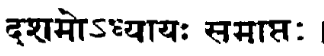End of chapter 10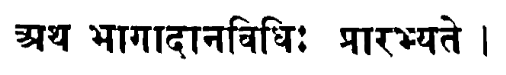Start of chapter 11: factoring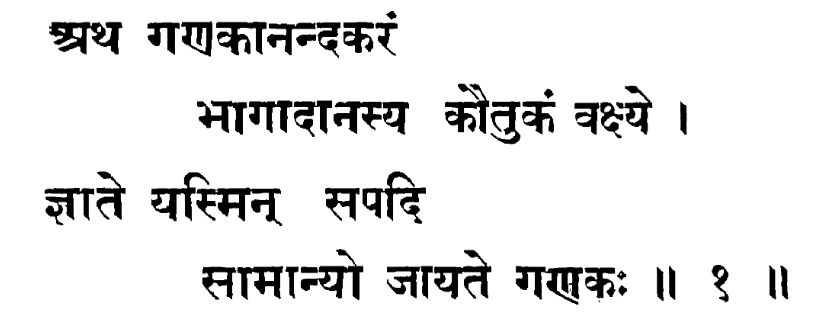I shall tell you of the delight of mathematicians, the joy of factoring. Learning this turns anyone into a mathematician.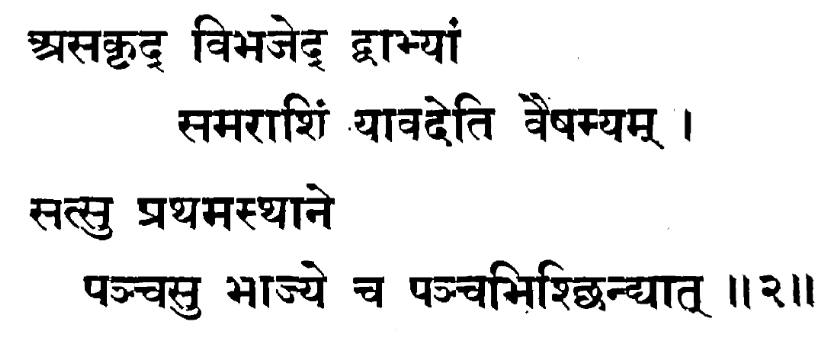Repeatedly divide an even number by 2, until it becomes odd. If it (the number to be factored) has 5 in the first [units] place, divide by 5.
• asakṛt = repeatedly
• sama = even; viṣama = odd
• In the Sanskrit tradition, numbers were (spoken and) written little-endian, i.e. units place first.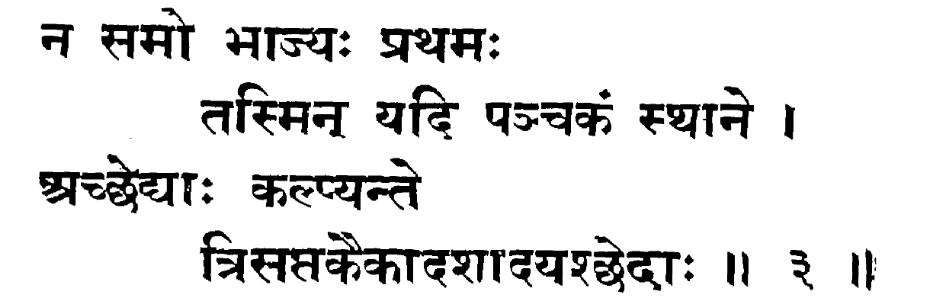When $N$ is not even nor has $5$ in the units place, try the prime numbers—3, 7, 11, etc—as divisors.
• acchedya = prime number (lit. indivisible)
• tri, saptaka, ekādaśa = 3, 7, 11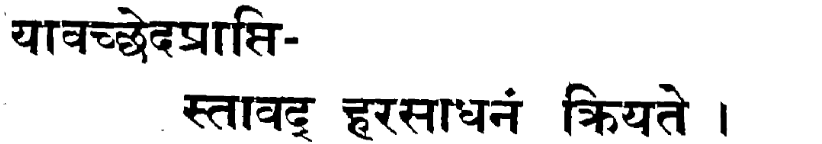While you find divisors, keep finding divisors.
hara = divisor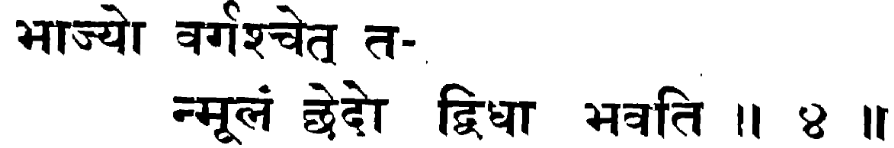If $N$ is a square, then its root is a factor, twice.
[An alternative method starts here…]
• varga = square
• mūla = root (literally! Maybe in English the mathematical sense came from elsewhere?)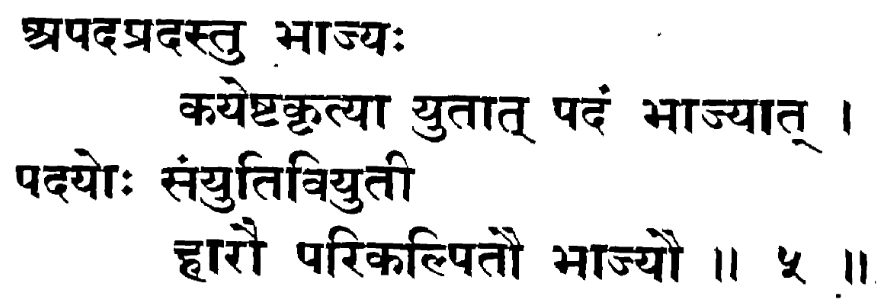When $N$ is not a square, when added to some square it becomes a square. Then, the sum and difference of the two square-roots are factors.
That is, there exists some square $s^2$ such that $N + s^2$ is a square, say $N + s^2 = b^2$. Then $b - s$ and $b+ s$ are factors of $N$.
• apadaprada = not square (lit. does not give a square root)
• iṣṭa = assumed / unknown
• kṛti = square
• saṃyuti = sum
• viyuti = difference
• hāra = divisor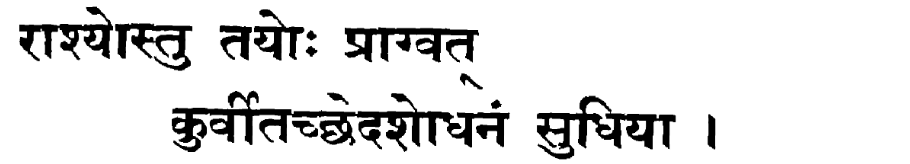Of these numbers, as before, find factors.
That is, factorize the $b-s$ and $b+s$ further.
rāśi = quantity, number — a number that has been written down, e.g. an output of a previous step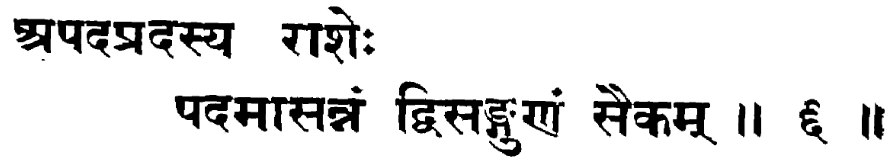Of a non-square number, find the nearest (integer) square root, double it and add 1…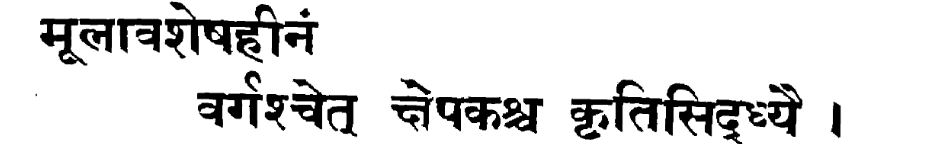…and subtract from it the square-remainder. If the result is a square, then this is the number to be added.
That is, let $N = m^2 + r$. Then if $2m + 1 - r$ is a square say $s^2$, then $N + s^2$ is $(m^2 + r) + (2m + 1 - r)$ which is $(m + 1)^2$, so we are done, as we have found a square $s^2$ such that $N + s^2$ is a square.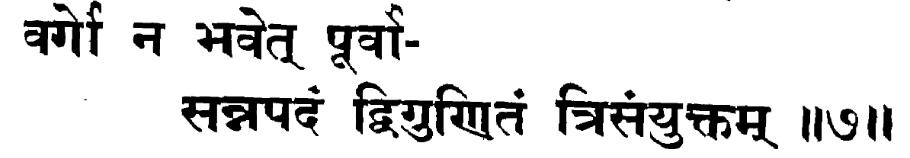If it is not a square, then further add $2m + 3$…
The idea is that we’re trying successive values for the smaller square: if $(m + 1)^2 - N$ isn’t a square, then the next option would be to replace $(m + 1)^2$ with $(m + 2)^2$, which is $(m + 1)^2 + (2m + 3)$.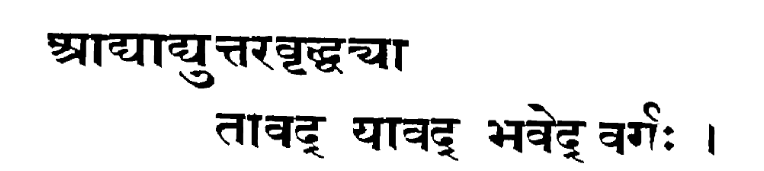…and so on in arithmetic progression, until you get a square.
So to $2m + 1 - r$, we’re adding $2m+3$, then $2m+5$ and $2m+7$ and so on. This way, we’re trying to write $N = a^2 - s^2$ by trying, for $a$, successive values $m+1$ then $m+2$ and so on until we find a square $b^2$, but without having to square each time. (We do need to test squareness each time, though.)
I think uttara = difference and uttaravṛddhi = arithmetic progression.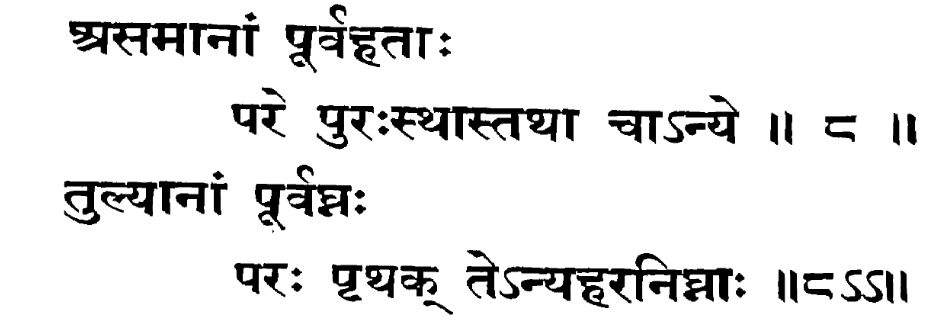For unequal divisors, put the previous products in front of them, and again separately. For equal divisors, put their products with each of the previous products, and again separately.
This is a procedure to list all divisors of a number.
• For example, say $N = 2 \times 3 \times 5 \times 7 \times 7$.
• Then, write down the list divisors = 
• Then append $d \times 3$ for each divisor $d$ in the list currently, and also append $3$ itself. The list becomes: [2, 2×3, 3].
• Then append $d \times 5$ for each divisor $d$ in the list currently, and also append $5$ itself. The list becomes: [2, 2×3, 3, 2×5, 2×3×5, 3×5, 5].
• Now for the two $7$s which are equal divisors, append $d \times 7$ and $d \times 7 \times 7$ for each divisor $d$ in the list currently, and also append $7$ and $7 \times 7$ themselves separately.
• This should give all divisors of $N$ (other than $1$, evidently).
Took me a while to understand but I think the anvaya is:
• pūrvahatāḥ asamānāṃ pare purasthāḥ, tathā ca anye = the prior products, in front of the unique [divisors], and also separately.
• pūrvaghnaḥ tulyānāṃ paraḥ, pṛthak, te anyaharanighnāḥ = the prior products in front of the equal [divisors], and separately, and they [the equal divisors] multiplied by the other (equal?) divisors.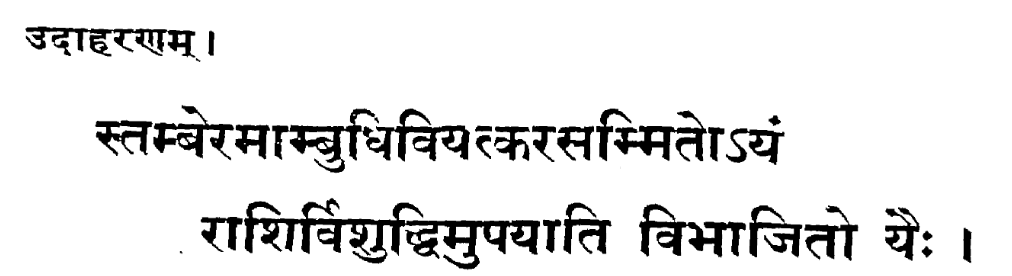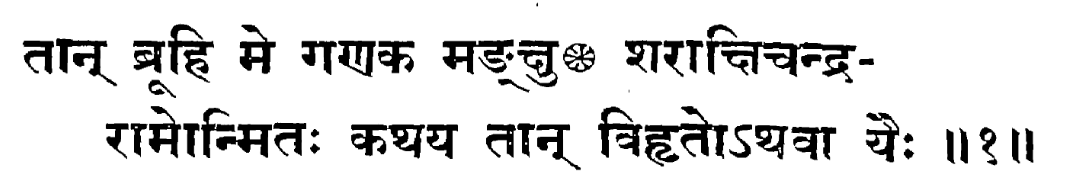Quickly find the divisors of 2048 and 3125.
Examples (exercises). Solutions:
• Solutions
• For 2048, repeatedly dividing by 2 gives it to be a product of eleven $2$s. We write them down: [2, 2, 2, 2, 2, 2, 2, 2, 2, 2, 2] and their products [2, 4, 8, 16, 32, 64, 128, 256, 512, 1024, 2048].
• For 3125, repeatedly dividing by 5 gives it to be a product of five $5$s. (Etc).
• Lit: viśuddhim upayāti vibhājito yaiḥ = those numbers on division by which it leaves zero remainder, i.e. its divisors.
• On the number names:
• stamberama-ambudhi-viyat-kara = 8-4-0-2 = 2048 (stamberama = elephant; sammita = “measured”)
• śara-akṣi-candra-rāma = 5-2-1-3, or 3125. (unmita = "having the measure of")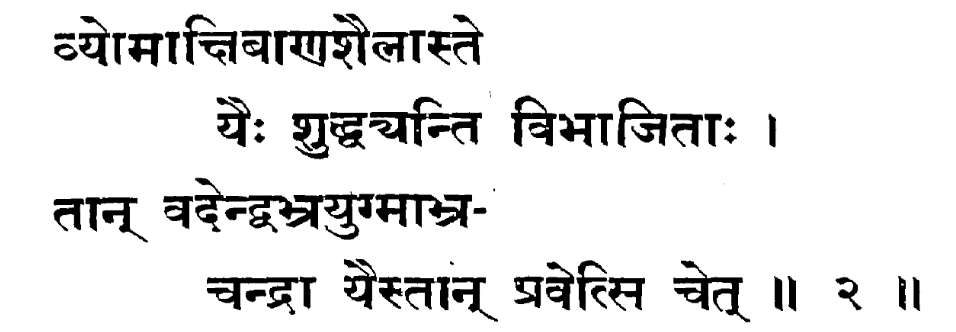Find the divisors of 7250, and those of 10201.
Solutions: It turns out that $7520 = 2^5 \times 5 \times 47$, and $10201 = 101^2$.
• vyoma-akṣi-bāṇa-śaila = 0-2-5-7 = 7520
• indu-abhra-yugma-abhra-candra = 1-0-2-0-1 = 10201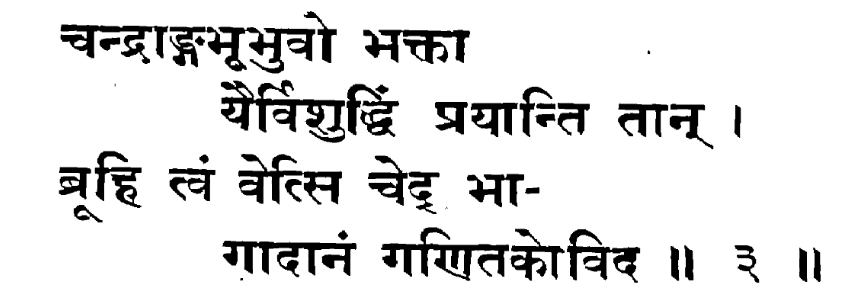List the divisors of 1161, if you’re a mathematician who knows how to factor.
Solutions: It turns out that $1161 = 3^3 \times 43$, which we could also get alternatively with $1161 = 34^2 + 5$ and $2\times34 + 1 - 5 = 64$ which is a square, so $1161 = 35^2 - 8^2 = 27 \times 43$.
candra-aṅga-bhū-bhuvaḥ = 1-6-1-1 = 1161.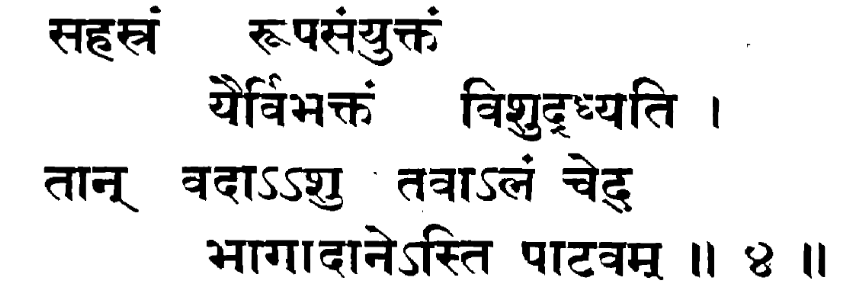The divisors of 1001 — name them quickly, if you have enough skill in factorization.
Solutions: The editor and translator use the second method and basically try lots of squares, but we can just use the first method (trial division) for $1001 = 7 \times 11 \times 13$, no?
sahasraṃ rūpa-saṃyuktaṃ = 1000 + 1 = 1001.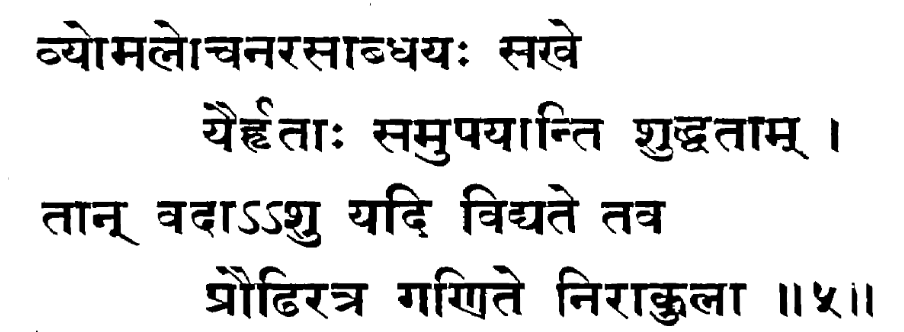The factors of 4620 — name them quickly, if you have complete proficiency in mathematics.
Solutions: $4620 = 2^2 \times 3 \times 5 \times 7 \times 11$. So there are 48 factors (or 47 without the 1). Again the editor factorizes 4620 by first removing the factors of 2 and 5 and then factorizing $3 \times 7 \times 11 = 231$ using the difference-of-squares method… but why?!
vyoma-locana-rasa-abdhayaḥ = 0-2-6-4 = 4620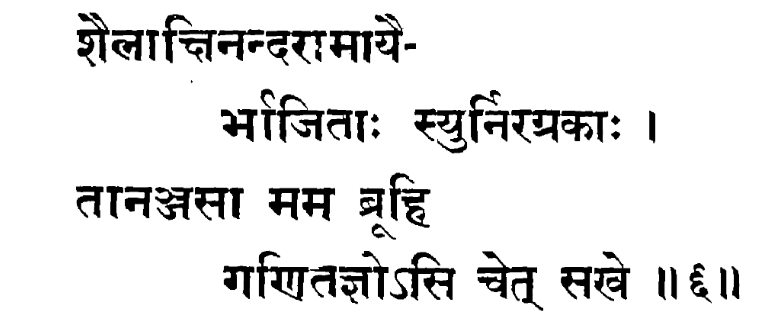Factor 3927.
Solutions: $3927 = 3 \times 7 \times 11 \times 17$.
śaila-akṣi-nanda-rāma = 7-2-9-3 = 3927.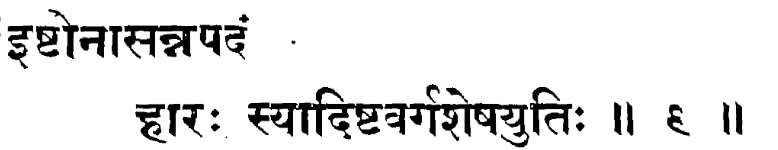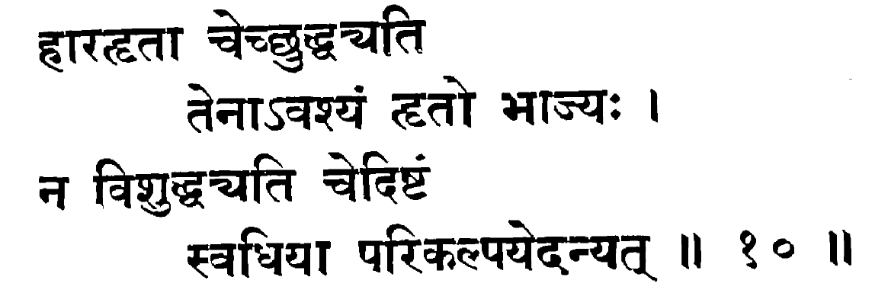• The nearest square-root, subtracted by some chosen number, is a divisor. If the chosen number when squared and added to the square-remainder…
• …is divisible by this divisor, then surely this divisor is a divisor of the original number.
• If it is not divisible, use your ingenuity to find a different choice.

[A third method starts here.]

That is, if $N = m^2 + r$, then for some unknown $x$, it is the case that $m - x$ divides $N$. (We want to find $x$.) If we find an $x$ such that $x^2 + r$ is divisible by $m - x$, then we have found $m - x$ as a factor of $N$. (This is actually nontrivial!)

In modern terms, the idea is this: write $N = m^2 + r$, and we’re trying to find some $p = m-x$ that divides $N$. Then, $m = p + x \equiv x \pmod p$, and so $N = m^2 + r \equiv x^2 + r \pmod p$. So we want $p = m - x$ to divide $x^2 + r$. That’s the rule.

That weird glyph that looks like tdṛ = त्दृ is actually is hṛ = हृ :-)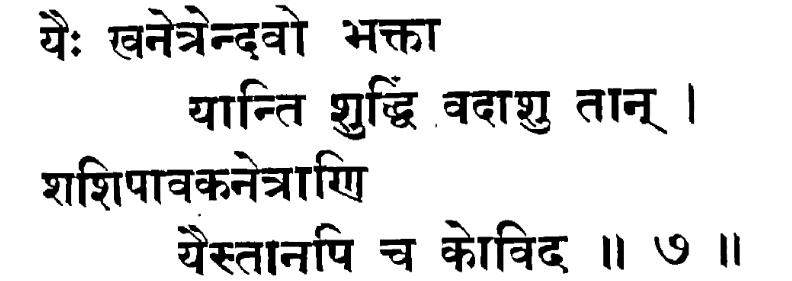Factors of 120. Factors of 231.
Solution (if we use this third method):
• $120 = 10^2 + 20$, and we want $10 - x$ to divide $x^2 + 20$. We can take $x = 2$, to see that $8$ divides $24$. Similarly, taking $x = 4$ gives $6$ (dividing $36$), and taking $x = 5$ gives $5$ (dividing $45$), and taking $x = 6$ gives $4$ (dividing $56$), and so on. More importantly, note that taking $x = 3$ shows that $7$ does not divide $3^2 + 20 = 29$, so $7$ is not a divisor of $120$.
• For $231 = 15^2 + 6$, we want $15 - x$ to divide $x^2 + 6$. Taking $x=4$ gives $11$ dividing $22$, so $11$ is also a divisor of $231$. Indeed, $231 = 3 \times 7 \times 11$.
kha-netra-indu = 0-2-1 = 120. śaśi-pāvaka-netra = 1-3-2 = 231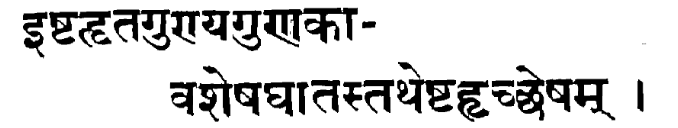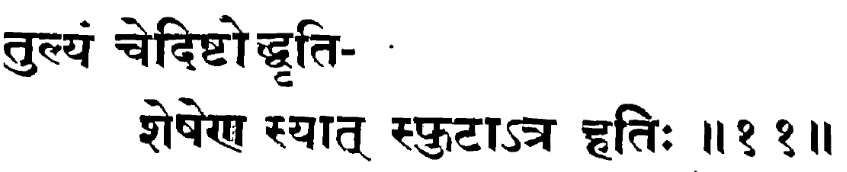By a chosen number, take remainders of both numbers being multiplied. Multiply them, and take remainder modulo the chosen number. If this remainder is the same (as that of the product), then the multiplication is [apparently] correct.
This is a “sanity check” for verifying a calculation: it’s saying that if you have a calculation $a \times b = c$ and you check that $(a \bmod m) \times (b \bmod m) \equiv (c \bmod m)$, then likely the calculation is correct.
hati = multiplication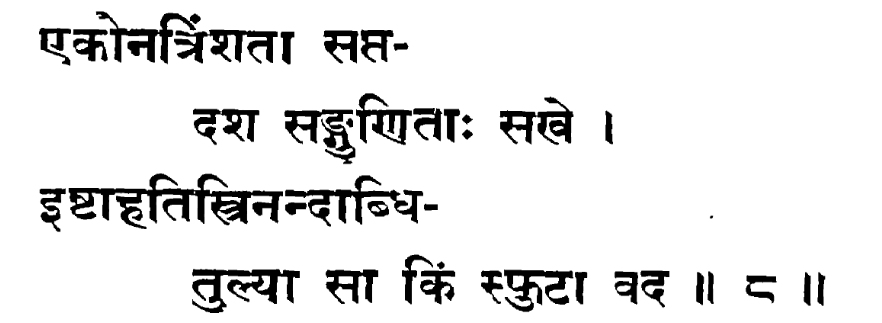$29 \times 17 = 493$ — check this.
The editor does the checking mod 3, 5, and 8: mod 3, we can see $2 \times 2 \equiv 4$. Mod 5, we can see $4 \times 2 \equiv 3$. Mod 8, we can see $5 \times 1 \equiv 5$. The calcuation is likely correct. (In modern terms, we could conclude that the multiplication is correct mod $120$, by the Chinese Remainder Theorem.)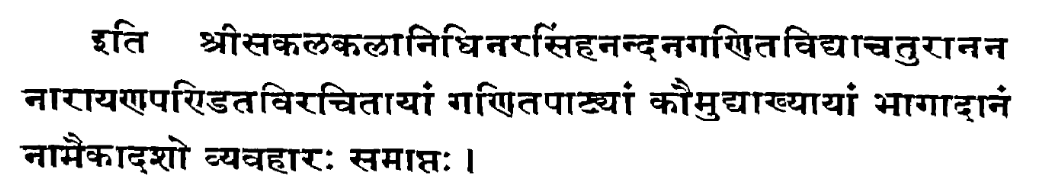Here ends vyavahāra (chapter) 11, named bhāgādāna (factorization), in the gaṇita text named Kaumudī, by Nārāyaṇa, who is “sakala-kalā-nidhi narasiṃha-nandana” (son of Narasiṃha), and “gaṇita-vidyā-caturānana”.

So to summarize, the mathematical content of the chapter is:

• Three methods for factoring numbers:
• Trial division by primes: Divide out factors of $2$ and $5$, then try dividing $N$ by $3$, $7$, $11$, $13$ etc.
• If $N = m^2 + r$, then to $2m - 1 + r$ until you get a square try adding $2m + 3$, $2m + 5$ etc. When we get a square $s^2$, then $N + s^2 = b^2$ will also be a square, and $b - s$ and $b + s$ are factors of $N$.
• If $N = m^2 + r$, find some $x$ such that $m - x$ divides $x^2 + r$. Then $m - x$ is a factor of $N$.
• An algorithm for enumerating all divisors of $N$, from a collected list of prime divisors.
• A verification / sanity check procedure for multiplication that generalizes casting out nines, namely to do it modulo some number.

and exercises for each of them. The second method of course is what we call Fermat’s method, named after Pierre de Fermat who independently rediscovered and first described it in a letter in 1643 CE (he must have discovered it some time after 1638):

An odd number not a square can be expressed as the difference of two squares in as many ways as it is the product of two factors, and if the squares are relatively prime the factors are. But if the squares have a common divisor $d$, the given number is divisible by $\sqrt{d}$. Given a number $n$, for examples $2027651281$, to find if it be prime or composite and the factors in the latter case. Extract the square root of $n$. I get $r = 45029$, with the remainder $40440$. Subtracting the latter from $2r + 1$, I get $49619$, which is not a square in view of the ending $19$. Hence I add $90061 = 2+ 2r+ 1$ to it. Since the sum $139680$ is not a square, as seen by the final digits, I again add to it the same number increased by $2$, i.e., $90063$, and I continue until the sum becomes a square. This does not happen until we reach $1040400$, the square of $1020$. For by an inspection of the sums mentioned it is easy to see that the final one is the only square (by their endings except for $499944$). To find the factors of $n$, I subtract the first number added, $90061$, from the last, $90081$. To half the difference add $2$. There results $12$. The sum of $12$ and the root $r$ is $45041$. Adding and subtracting the root $1020$ of the final sum $1040400$, we get $46061$ and $44021$, which are the two numbers nearest to $r$ whose product is $n$. They are the only factors since they are primes. Instead of $11$ additions, the ordinary method of factoring would require the division by all the numbers from $7$ to $44021$.

• This Gaṇita-kaumudī was written in 1356 CE (as mentioned above), about 300 years before Fermat came up with the method, and a further 300 years earlier, the author Śrīpati in his Siddhāntaśekhara (1039 CE) gives a limited form of the method: if $N = m^2 + r$ and it so happens that $2m + 1 - r$ is a square say $b^2$, then $N = (a + 1)^2 - b^2$ giving the two factors $a + 1 - b$ and $a + 1 + b$. So Nārāyaṇa can be seen as turning this idea into an actual method for factoring. (Reference: K. S. Shukla, Hindu methods for fnding factors or divisors of a number, Gaṇita, Vol. 17, No. 2 (1966), pp. 109–117, reprinted in “Studies in Indian Mathematics and Astronomy: Selected Articles of Kripa Shankar Shukla” edited by Aditya Kolachana, K. Mahesh, K. Ramasubramanian, Hindustan Book Agency / Springer (2019): https://doi.org/10.1007/978-981-13-7326-8_13)

• To me this example shows that even in the less-notable parts of works, there can be interesting stuff. (The Gaṇita-kaumudī is much more notable for its combinatorics and study of magic squares, and even in the arithmetic parts there are more interesting things like generalizations of Pell’s equation etc.)

• Fermat was working with 10-digit numbers for which trial division is clearly a lot more work, but it is not clear to me whether Nārāyaṇa had any need for his method (whether he was factoring such large numbers), or it just came about as the result of generally thinking about numbers and factoring. (That is, it is not clear whether the small examples he gives were small just for the sake of exposition.)

• The third method of Nārāyaṇa is not an explicit algorithm, given the “use your ingenuity” part, and it seems much less useful as a factoring method for large numbers, but I think the underlying idea still has some value / relation to some of the ideas in modern factoring algorithms like the quadratic sieve. I’ll think more about this while writing a future planned post on Cole/Seelhoff etc.

• Given the huge importance of this work of Nārāyaṇa, you might imagine that there are several manuscripts of this work carefully preserved in several libraries, etc. Alas, the truth is that, as far as I can tell (NCC), all that remain today is two manuscripts of Chapters 13–14, and the text printed in two volumes in 1936 and 1942. The story of the printed edition is this, quoting Padmakara Dvivedi Jyautiśācārya who edited and printed it:

After the death of my revered father [Mahāmahopādhyāya Pandit] Sudhakara Dvivedi, I discovered a complete manuscript of this work in his collection. I immediately set to work upon it…

and (from the first volume)

श्रीपूज्यपादपितृसंगृहीतपुस्तकेषु तेषां निधनात्पश्चान्मया श्रीनृसिंहनन्दननारायणपण्डितरचिताया गणितकौमुद्या एकाशुद्धा हस्तलिखतप्रतिः प्राप्ता। लुप्तप्रायस्य सुतरां कुत्राप्यमुद्रितपूर्वस्य गणितकौमुदीग्रन्थस्यायं प्रथमप्रकाशनावसरः।

(śrī-pūjyapāda-pitṛ-saṃgṛhīta-pustakeṣu teṣāṃ nidhanāt paścān mayā śrī-nṛsiṃha-nandana-nārāyaṇa-paṇḍita-racitāyā gaṇita-kaumudyā ekāśuddhā hasta-likhata-pratiḥ prāptā. lupta-prāyasya sutarāṃ kutrāpy amudrita-pūrvasya gaṇita-kaumudī-granthasyāyaṃ prathama-prakāśanāvasaraḥ.)

So consider all the things that had to go right, for this work not to be lost:

• Pandit Sudhakara Dvivedi had a copy of the manuscript in his collection,

• His son Padmakara Dvivedi (Jyautiśācārya) was also a scholar in the same tradition,

• He happened to recognize the manuscript and its importance, and had the energy and ability to edit it and print it (with his commentary/notes),

• There was a publisher at the time (1936 and 1942) that considered the work worth printing.

• Enough libraries carried copies of this printed book that scans of it are available to us today.

And this is a pretty much a “best case” scenario. How many other manuscripts were lost, as traditional learning declined, or children were not up to publishing works from their parents' collections, or could not find a publisher? As it is, the printed version is based on a single complete manuscript (though the preface to the first volume mentions another manuscript from Nepal, found after printing), which for all I can tell may not even be available any more. This is the fate of one of the major works of Indian mathematics. See also the article The Untapped Wealth Of Manuscripts On Indian Astronomy And Mathematics by M. D. Srinivas (here / here). (Maybe there’s hope: there’s a listing in the National Mission for Manuscript’s database, so maybe a manuscript still exists after all?)

• As mentioned above, the work has been printed, and there are some scans of this work online:

• An English translation (based on the printed book) was published by Paramanand Singh across several issues of the Gaṇita-bhāratī journal (Bull. Ind. Soc. Hist. Math.) during 1998–2002:

• 20 (1998): 25–82 (Chaps I–III);
• 21 (1999): 10–73 (Chap. IV);
• 22 (2000): 19–85 (Chaps V–XII);
• 23 (2001): 18–82 (Chap XIII); and
• 24 (2002): 35–98 (Chap. XIV).

The journal is hard to find but someone has kindly compiled the translation of Chapters 1 to 13 and uploaded the translation here (translation of Chap 14 is mising).

• There are three lectures on the Gaṇita-kaumudī in the NPTEL course on “Mathematics in India - From Vedic Period to Modern Times” (YouTube playlist):

• Many sources mention that the Gaṇita-kaumudī comes with both a mūla (source) text and a vāsanā (commentary) written by Nārāyaṇa himself; the latter would be very valuable and it’s not clear to me whether the latter has been published or is available anywhere.